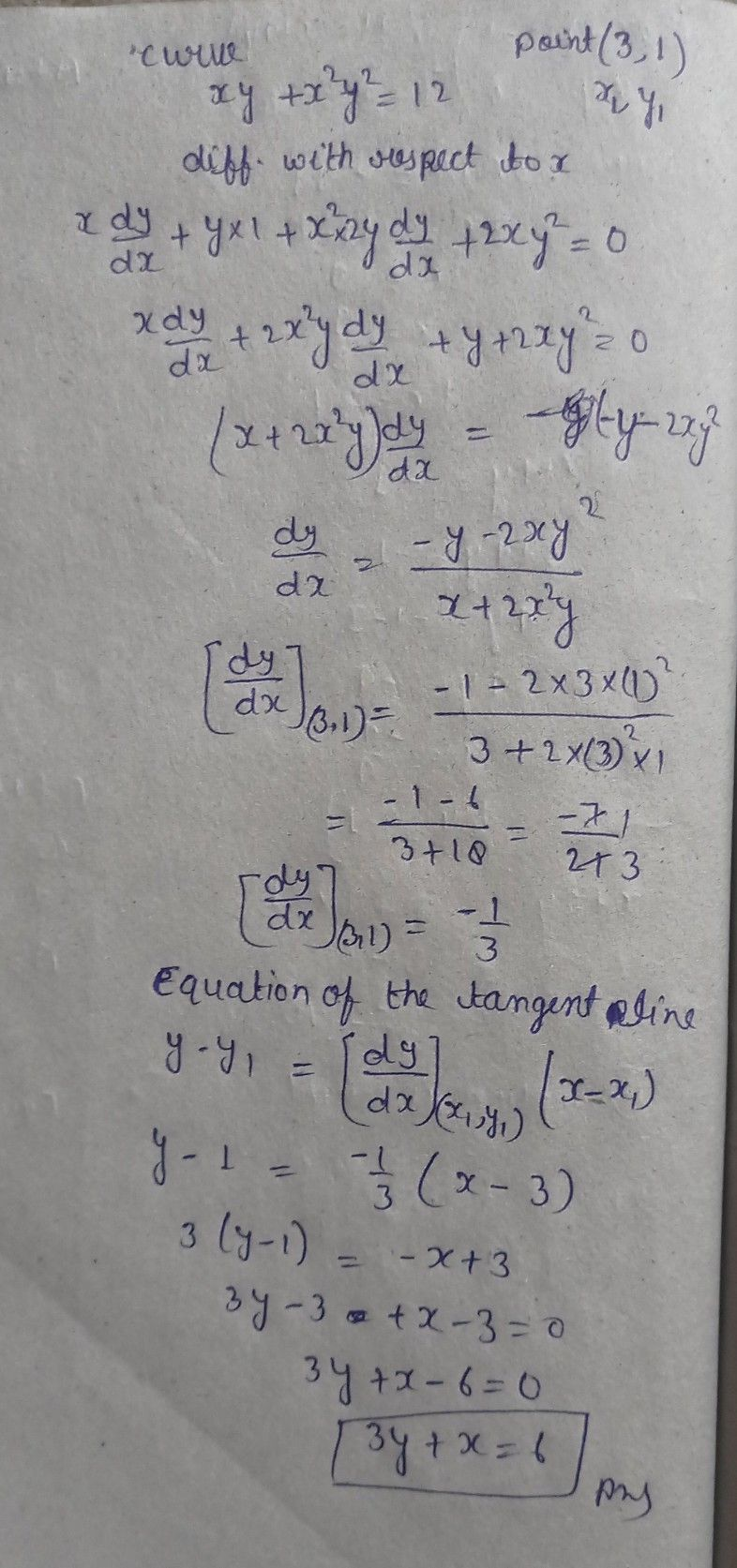Symbol
ProblemFind the equation of the tangent line to the curve $xy+x^{2}y^{2}=12$ at the point $\left(3,1\right)$
Calculus
Search count: 107
SolutionQanda teacher - Anshulplease give me review by 5 ?